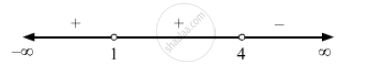Advertisement Remove all ads

# Write the set of values of x satisfying the inequation (x2 − 2x + 1) (x − 4) < 0. - Mathematics

Write the set of values of x satisfying the inequation (x2 − 2x + 1) (x − 4) < 0.

Advertisement Remove all ads

#### Solution

We have:

$\left( x^2 - 2x + 1 \right)\left( x - 4 \right) < 0$
$\Rightarrow (x - 1 )^2 (x - 4) < 0$
$\text{ Equating each one to zero, we obtain } x = 1 \text{ and } x = 4 .$
$\text{ Therefore, 1 and 4 are critical points } .$
$\text{ Drawing the number lines, we get }:$$\text{ Therefore, the solution set of the given inequality is x } \in \left( - \infty , 1 \right) \cup \left( 1, 4 \right)$

Is there an error in this question or solution?
Advertisement Remove all ads

#### APPEARS IN

RD Sharma Class 11 Mathematics Textbook
Chapter 15 Linear Inequations
Q 3 | Page 31
Advertisement Remove all ads

#### Video TutorialsVIEW ALL 

Advertisement Remove all ads
Share
Notifications

View all notifications

Forgot password?